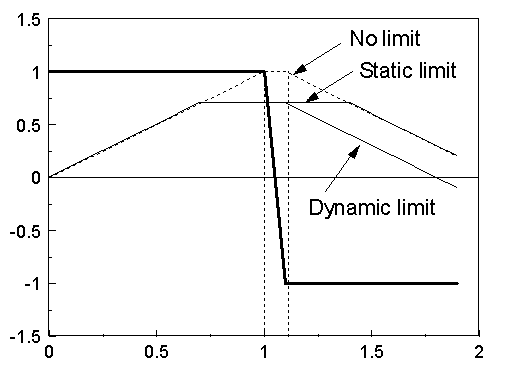﻿ MODELS > Examples > Models Limits

# Models Limits

Limits on expressions are generally specified {min:a max:b}

Limits are generally used to restrict variables inside certain ranges.

The first example illustrates constraints on derivatives.

MODEL derivlim

CONST freq {val: 50}

omega {val: 2*pi*freq}

half_period {val: 0.5/freq}

VAR x       -- applied signal

dx     -- derivative of x

dxlim   -- derivative of x with limits

y       -- integral of dxlim

INIT

histdef(x)     :=0;             -- needed for calculation of deriv()

histdef(dxlim) :=0;             -- needed for calculation of integral()

histdef(integral(dxlim)) :=0;   -- needed for calculation of integral()

ENDINIT

EXEC

x:= (1 -cos(omega*t))*(t<=3*half_period) -- active until t=half_period

+ 2*(t>3*half_period)                 -- active after t=half_period

dx:= deriv(x)

dxlim := dx {max: 0.6*omega} -- limits the derivative

y:= integral(dxlim) -- reconstructs signal with limit applied on derivative

ENDEXEC

ENDMODEL

The next example illustrates dynamic limit on integral in MODELS.

Only dynamic limits are allowed directly on integral {dmin:a dmax:b}

A static limits can be set on the integral value after evaluation of the integral.

MODEL exa_integral

VAR x, y   -- step signal =1 from t=0 to t<=1, =-1 for t>1

dlim   -- integral(x), dynamic limit=0.7

nolim -- integral(y), no limit

slim   -- static limit=0.7 applied to 'nolim'

HISTORY x {dflt: 0}

y {dflt: 0}

integral(x) {dflt: 0}

integral(y) {dflt: 0}

EXEC

x:=  1

IF t>1 THEN

x:= -1

ENDIF

y:= x

dlim  :=integral(x){dmax: 0.7}

nolim :=integral(y)

slim  :=nolim {:0.7}

ENDEXEC

ENDMODEL

The third example illustrates dynamic limit on lapace/claplace in MODELS.

Only dynamic limits are allowed directly on laplace {dmin:a dmax:b}, placed before the transfer function.

A static limits can be set on the output value after evaluation of the transfer function.

MODEL exa_laplace

DATA    Vdcref {DFLT:200}       -- Required DC-Link Voltage [V]

Vdcini {DFLT:690}       -- Initial amplitude of the DC Voltage [V]

INPUT   Vsup, Vinf

OUTPUT  Vdc

VAR     K_P, tau_P, Vdc, ErrV, Err_V, Vdc

HISTORY Err_V {dflt:0}

HISTORY Vdc   {dflt:Vdcini}

INIT

K_P := 1.5

tau_P := 0.05/K_P

ENDINIT

EXEC

ErrV := Vdcref-(Vsup-Vinf)

LAPLACE(Err_V/ErrV) := (1|S0)/(1|S0+0.03|S1)

LAPLACE(Vdc/Err_V) {dmin:0. dmax:2000.} := (1|S0+(K_P*tau_P)|S1)/(0|S0+tau_P|S1)

ENDEXEC

ENDMODEL

A dynamic limit means that the derivative of the output is set to zero when the unrestricted output is outside the limits. For integrals this means that the integrand is set to zero as long as its contribution causes the output to break the limits. In the example above the integrand in integral(x) x is set to zero when dlim is larger than 0.7 and x>0 (positive contribution to the integral).

A static limit is the most normal case and applies directly on the unlimited variable.

Fig. 1 shows the result of ilim model above. x is drawn as a thick line and nolim, slim and dlim indicated as Nolimit, static limit and dynamic limit respectively.Fig. 1. Results of the model ilim.